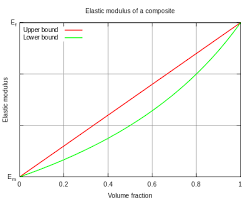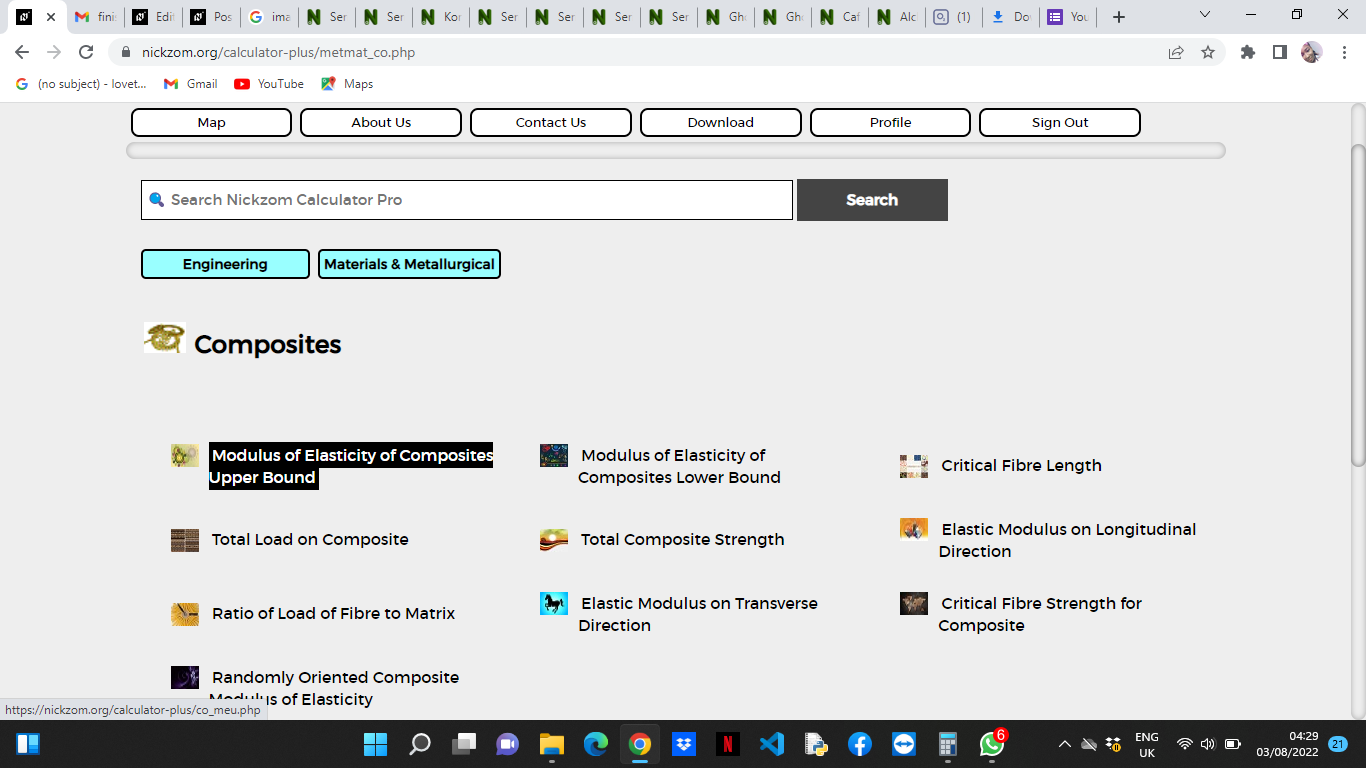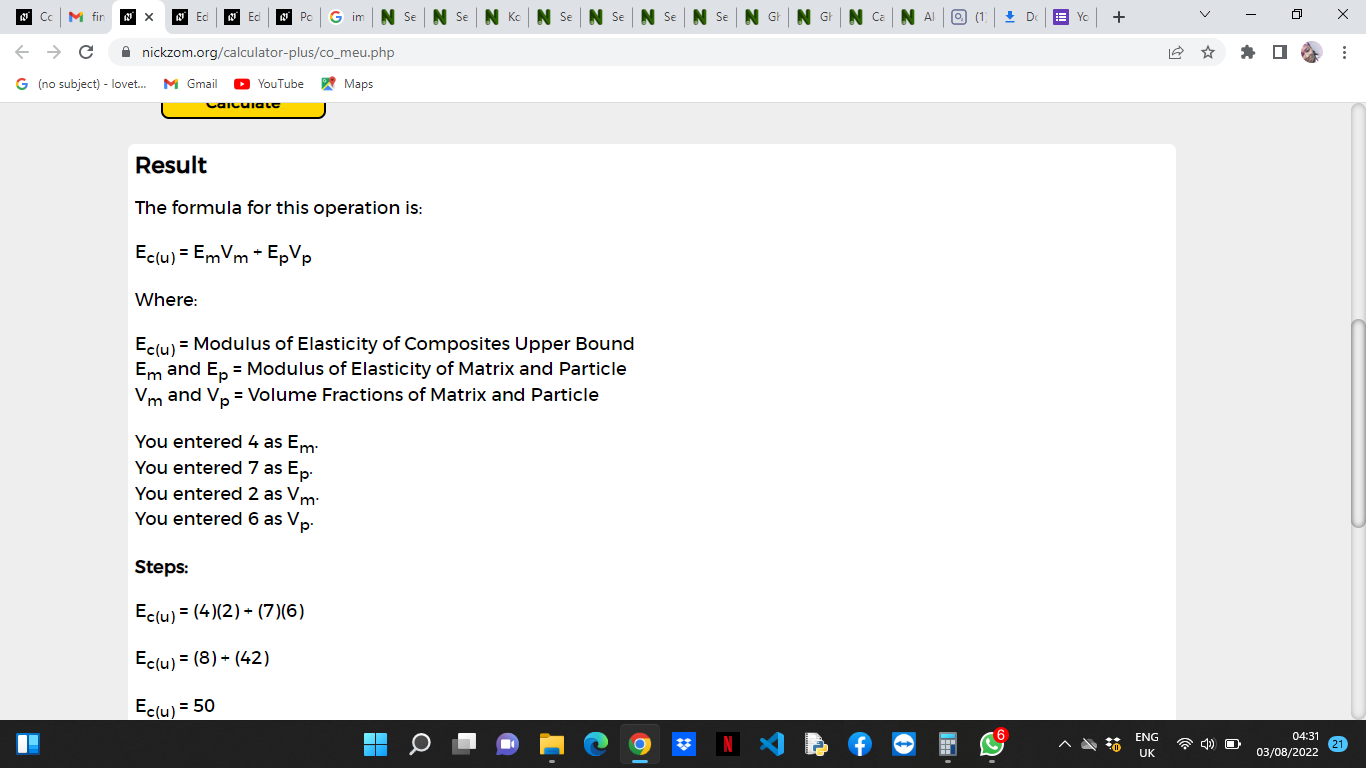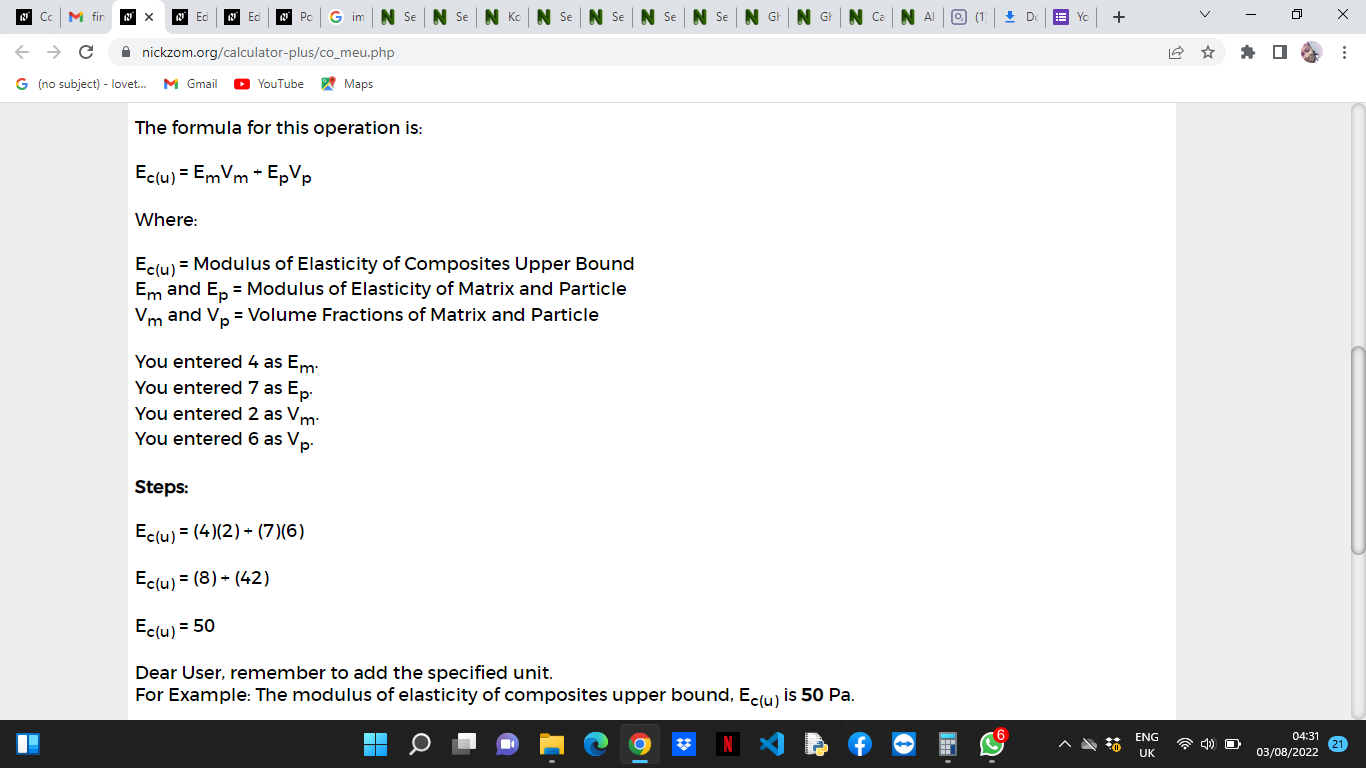# How to Calculate and Solve for Modulus of Elasticity of Composites Upper Bound | Composites

The modulus of elasticity of composites upper bound is illustrated by the image below.To compute for modulus of elasticity of composites upper bound, four essential parameters are needed and these parameters are Modulus of Elasticity of the Matrix (Em), Modulus of Elasticity of the Particle (Ep), Volume Fraction of the Matrix (Vm) and Volume Fraction of the Particle (Vp).

The formula for calculating modulus of elasticity of composites upper bound:

Ec(u) = EmVm + EpVp

Where:

Ec(u) = Modulus of Elasticity of Composites Upper Bound
Em =Modulus of Elasticity of the Matrix
Ep = Modulus of Elasticity of the Particle
Vm = Volume Fractions of the Matrix
Vp = Volume Fractions of the Particle

Let’s solve an example;
Find the modulus of elasticity of composites upper bound when the modulus of elasticity of the matrix is 4, the modulus of elasticity of the particle is 7, the volume fractions of the matrix is 2 and the volume fractions of the particle is 6.

This implies that;

Em =Modulus of Elasticity of the Matrix = 4
Ep = Modulus of Elasticity of the Particle = 7
Vm = Volume Fractions of the Matrix = 2
Vp = Volume Fractions of the Particle = 6

Ec(u) = EmVm + EpVp
Ec(u) = (4)(2) + (7)(6)
Ec(u) = (8) + (42)
Ec(u) = 50

Therefore, the modulus of elasticity of composites upper bound is 50 Pa.

Calculating the Modulus of Elasticity of the Matrix when the Modulus of Elasticity of Composites Upper Bound, the Modulus of Elasticity of the Particle, the Volume Fraction of the Matrix and the Volume Fraction of the Particle is Given.

Em = Ec(u) – EpVp / Vm

Where:

Em =Modulus of Elasticity of the Matrix
Ec(u) = Modulus of Elasticity of Composites Upper Bound
Ep = Modulus of Elasticity of the Particle
Vm = Volume Fractions of the Matrix
Vp = Volume Fractions of the Particle

Let’s solve an example;
Find the modulus of elasticity of the matrix when the modulus of elasticity of composites upper bound is 40, the modulus of elasticity of the particle is 10, the volume fractions of the matrix is 5 and the volume fractions of the particle is 2.

This implies that;

Ec(u) = Modulus of Elasticity of Composites Upper Bound = 40
Ep = Modulus of Elasticity of the Particle = 10
Vm = Volume Fractions of the Matrix = 5
Vp = Volume Fractions of the Particle = 2

Em = Ec(u) – EpVp / Vm
Em = 40 – (10)(2) / 5
Em = 40 – 20 / 5
Em = 20 / 5
Em = 4

Therefore, the modulus of elasticity of the matrix is 4.

Calculating the Modulus of Elasticity of the Particle when the Modulus of Elasticity of Composites Upper Bound, the Modulus of Elasticity of the Particle, the Volume Fraction of the Matrix and the Volume Fraction of the Particle is Given.

Ep = Ec(u) – EmVm / Vp

Where:

Ep = Modulus of Elasticity of the Particle
Ec(u) = Modulus of Elasticity of Composites Upper Bound
Em =Modulus of Elasticity of the Matrix
Vm = Volume Fractions of the Matrix
Vp = Volume Fractions of the Particle

Let’s solve an example;
Find the modulus of elasticity of the particle when the modulus of elasticity of composites upper bound is 50, the modulus of elasticity of the matrix is 4, the volume fractions of the matrix is 5 and the volume fractions of the particle is 10.

This implies that;

Ec(u) = Modulus of Elasticity of Composites Upper Bound = 50
Em =Modulus of Elasticity of the Matrix = 4
Vm = Volume Fractions of the Matrix = 5
Vp = Volume Fractions of the Particle = 10

Ep = Ec(u) – EmVm / Vp
Ep = 50 – (4)(5) / 10
Ep = 50 – 20 / 10
Ep = 30 / 10
Ep = 3

Therefore, the modulus of elasticity of the particle is 3.

Calculating the Volume Fraction of the Matrix when the Modulus of Elasticity of Composites Upper Bound, the Modulus of Elasticity of the Matrix, the Modulus of Elasticity of the Particle and the Volume Fraction of the Particle is Given.

Vm = Ec(u) – EpVp / Em

Where:

Vm = Volume Fractions of the Matrix
Ec(u) = Modulus of Elasticity of Composites Upper Bound
Em =Modulus of Elasticity of the Matrix
Ep = Modulus of Elasticity of the Particle
Vp = Volume Fractions of the Particle

Given an example;
Find the volume fractions of the matrix when the modulus of elasticity of composites upper bound is 30, the modulus of elasticity of the matrix is 3, the modulus of elasticity of the particle is 5 and the volume fractions of the particle is 3.

This implies that;

Ec(u) = Modulus of Elasticity of Composites Upper Bound = 30
Em =Modulus of Elasticity of the Matrix = 3
Ep = Modulus of Elasticity of the Particle = 5
Vp = Volume Fractions of the Particle = 3

Vm = Ec(u) – EpVp / Em
Vm = 30 – (5)(3) / 3
Vm = 30 – 15 / 3
Vm = 15 / 3
Vm = 5

Therefore, the volume fraction of the matrix is 5.

Calculating the Volume Fraction of the Particle when the Modulus of Elasticity of Composites Upper Bound, the Modulus of Elasticity of the Matrix, the Modulus of Elasticity of the Particle and the Volume Fraction of the Matrix is Given.

Vp = Ec(u) – EmVm / Ep

Where:

Vp = Volume Fractions of the Particle
Ec(u) = Modulus of Elasticity of Composites Upper Bound
Em =Modulus of Elasticity of the Matrix
Ep = Modulus of Elasticity of the Particle
Vm = Volume Fractions of the Matrix

Let’s solve an example;
Find the volume fractions of the particle when the modulus of elasticity of composites upper bound is 10, the modulus of elasticity of matrix is 2, the modulus of elasticity of the particle is 4 and the volume fraction of the matrix is 3.

This implies that;

Ec(u) = Modulus of Elasticity of Composites Upper Bound = 10
Em =Modulus of Elasticity of the Matrix = 2
Ep = Modulus of Elasticity of the Particle = 4
Vm = Volume Fractions of the Matrix = 3

Vp = Ec(u) – EmVm / Ep
Vp = 10 – (2)(3) / 4
Vp = 10 – 6 / 4
Vp = 4 / 4
Vp = 1

Therefore, the volume fractions of the particle is 1.

Nickzom Calculator – The Calculator Encyclopedia is capable of calculating the modulus of elasticity of composites upper bound.

To get the answer and workings of the modulus of elasticity of composites upper bound using the Nickzom Calculator – The Calculator Encyclopedia. First, you need to obtain the app.

You can get this app via any of these means:

You can also try the demo version via https://www.nickzom.org/calculator

Apple (Paid) – https://itunes.apple.com/us/app/nickzom-calculator/id1331162702?mt=8
Once, you have obtained the calculator encyclopedia app, proceed to the Calculator Map, then click on Materials and Metallurgical under Engineering.Now, Click on Composites under Materials and MetallurgicalNow, Click on Modulus of Elasticity of Composites Upper Bound under CompositesThe screenshot below displays the page or activity to enter your values, to get the answer for the modulus of elasticity of composites upper bound according to the respective parameter which is the Modulus of Elasticity of the Matrix (Em), Modulus of Elasticity of the Particle (Ep), Volume Fraction of the Matrix (Vm) and Volume Fraction of the Particle (Vp).Now, enter the values appropriately and accordingly for the parameters as required by the Modulus of Elasticity of the Matrix (Em) is 4, Modulus of Elasticity of the Particle (Ep) is 7, Volume Fraction of the Matrix (Vmis 2 and Volume Fraction of the Particle (Vp) is 6.Finally, Click on CalculateAs you can see from the screenshot above, Nickzom Calculator– The Calculator Encyclopedia solves for the modulus of elasticity of composites upper bound and presents the formula, workings and steps too.